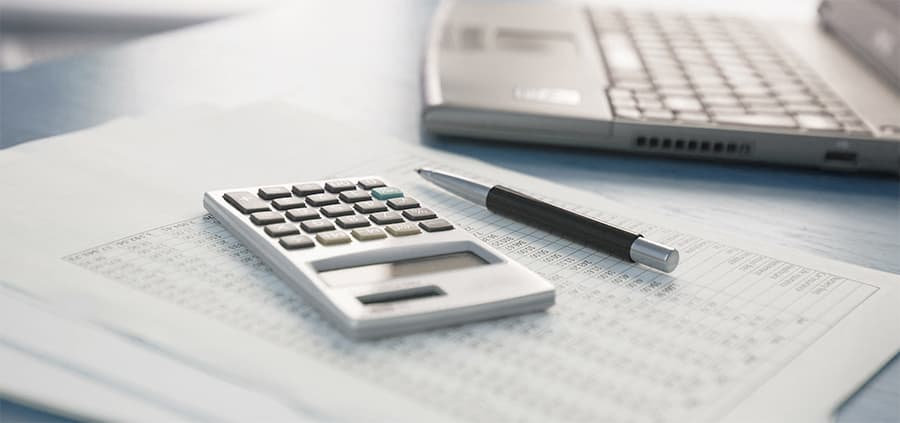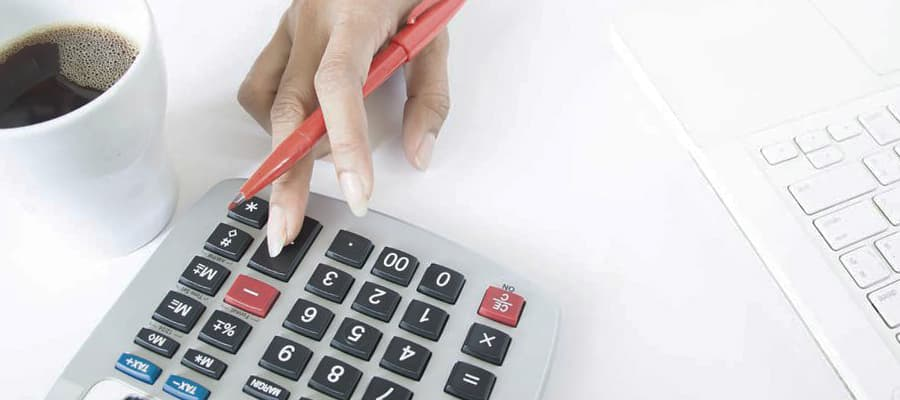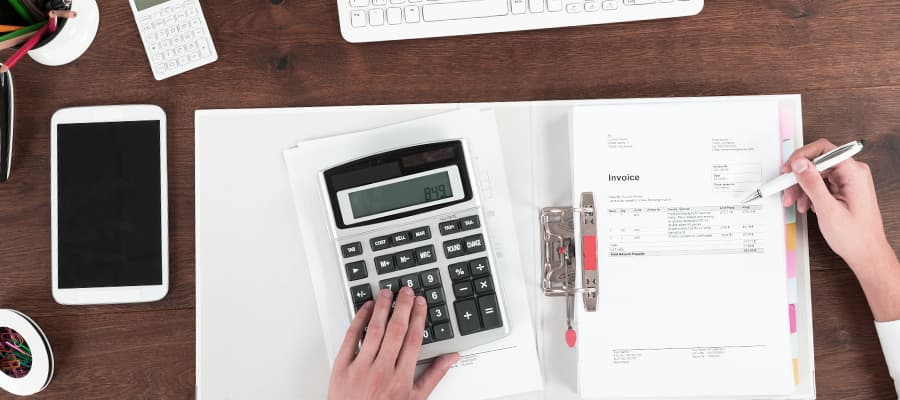# Simple Calculator

Simply calculate whatever you need.

Contents

## What is Simple Calculator and How Calculate it?A simple calculator is a handheld electronic device that performs basic arithmetic operations like addition, subtraction, multiplication, and division. It can also calculate percentages, square roots, and exponents. Modern calculators can also have more advanced features such as graphing and scientific calculations.

To use a simple calculator, you need to input the numbers and operations you want to perform using the buttons provided on the device. The standard layout of a calculator includes the numbers 0 through 9, decimal point, and arithmetic operators such as plus (+), minus (-), multiplication (*), and division (/).

For example, to add two numbers using a simple calculator, you would enter the first number, press the plus button, and then enter the second number. Finally, pressing the equals (=) button will display the result of the calculation.

Similarly, for other arithmetic operations, you would use the appropriate operator button to perform the calculation. Some calculators also have memory functions that allow you to store intermediate results for use in subsequent calculations.

Overall, a simple calculator is a handy tool for performing basic mathematical calculations quickly and accurately.

## How to Use a Simple Calculator?

Using a simple calculator is a straightforward process that can be broken down into the following steps:

Turn on the calculator. Most simple calculators have a button labeled "ON/C" or "AC". Press this button to turn on the calculator.

Familiarize yourself with the buttons. Look at the buttons on your calculator and familiarize yourself with their functions. The most common buttons on a calculator include the number buttons (0-9), the arithmetic operators (+, -, x, ÷), and the equals button (=). Other common buttons include the decimal point (.), the percentage (%), the clear button (C or CE), and the memory buttons (M+, M-, MR, MC).

Enter the numbers. To perform a calculation, enter the numbers using the number buttons. For example, if you want to add 2 and 3, press the number 2, then the plus (+) button, then the number 3.

Use the arithmetic operators: Once you have entered the numbers, use the appropriate arithmetic operator to perform the calculation. For example, to add 2 and 3, press the plus (+) button. To subtract 2 from 3, press the minus (-) button.

Use parentheses. If you need to perform a calculation that involves multiple operations, you can use parentheses to group the operations together. For example, to calculate 2 x (3 + 4), enter 2, then the multiplication (x) button, then open parentheses, enter 3, then the plus (+) button, enter 4, then close parentheses.

Use the percentage button. The percentage button is used to calculate percentages. For example, if you want to calculate 20% of 50, enter 50, then the percentage (%) button, then 20.

Use the clear button. If you make a mistake while entering numbers or performing a calculation, use the clear button (C or CE) to start over.

Use the memory buttons. If you need to store a number for later use or retrieve a stored number, use the memory buttons (M+, M-, MR, MC). For example, to store the number 5, enter 5, then press the M+ button. To retrieve the stored number, press the MR button.

Use the equals button. Once you have entered the numbers and arithmetic operators, press the equals (=) button to perform the calculation.

Turn off the calculator. When you are finished using the calculator, turn it off by pressing the "OFF" or "AC" button.

By following these steps, you should be able to use a simple calculator to perform a wide range of calculations.

## Examples of Calculating Simple CalculatorHere are three examples of a simple calculator performing calculations:

Suppose you want to add 24 and 36. To do this on a simple calculator, you would first press the "2" button, then the "4" button, then the "+" button, then the "3" button, and finally the "6" button. The display on the calculator should show "60," which is the sum of 24 and 36.

### Subtraction

Suppose you want to subtract 17 from 43. To do this on a simple calculator, you would first press the "4" button, then the "3" button, then the "-" button, then the "1" button, and finally the "7" button. The display on the calculator should show "26," which is the difference between 43 and 17.

### Multiplication

Suppose you want to multiply 8 by 9. To do this on a simple calculator, you would first press the "8" button, then the "x" or "*" button, then the "9" button. The display on the calculator should show "72," which is the product of 8 and 9.

## Calculation NuancesCalculating with a simple calculator is a fairly straightforward process, but there are a few nuances to keep in mind:

Order of operations. The order in which you perform calculations matters. Use the acronym "PEMDAS" to remember the correct order: Parentheses, Exponents, Multiplication and Division (performed left to right), and Addition and Subtraction (performed left to right).

Accuracy. Simple calculators have a limited number of digits they can display, so it's important to be aware of rounding errors. If you need more precision, consider using a scientific calculator.

Negative numbers. When performing calculations with negative numbers, pay attention to whether you're entering a negative number or subtracting a positive number. You may need to use parentheses to clarify your intent.

Decimal points. If you're working with decimals, be sure to enter the decimal point in the correct place. A misplaced decimal point can result in a wildly incorrect answer.

Memory functions. Most calculators have memory functions that allow you to store and recall values. Be aware of how to use these functions, and clear the memory before starting a new calculation if necessary.

Overall, it's important to double-check your work and make sure you're entering the correct values and using the correct order of operations.

## Simple Calculator vs Manual CalculationWhen it comes to calculations, the efficiency of a simple calculator versus manual calculation depends on the complexity of the problem and the skill of the person performing the calculation.

For simple arithmetic operations like addition, subtraction, multiplication, and division, a calculator is often more efficient and accurate than manual calculation. This is especially true for larger numbers or when performing repetitive calculations. Calculators can also handle more complex mathematical functions like logarithms, trigonometric functions, and exponential functions, which would be time-consuming to calculate manually.

However, for very simple calculations, such as adding or subtracting small numbers, manual calculation can be faster than using a calculator. Additionally, manual calculation allows for greater flexibility in terms of estimating and rounding numbers, which can be useful in certain situations.

Ultimately, the choice between using a calculator or manual calculation depends on the nature of the problem and the individual's personal preference and skill level. In general, a calculator is a more efficient tool for complex mathematical operations, while manual calculation may be faster for simple calculations.

And of course, it`s worth answering some questions that arise during the calculations.

### How do I use a simple calculator?

A simple calculator typically has a number pad, mathematical operations such as addition, subtraction, multiplication, and division, as well as other functions such as square root, percentage, and exponentiation. To use the calculator, simply input the numbers and operations in the order you want them to be calculated and press the equal button to get the result. It`s important to remember the order of operations (PEMDAS), which dictates the sequence in which mathematical operations should be performed.

### How accurate are simple calculators?

Simple calculators are designed to be highly accurate and reliable. They use advanced algorithms and mathematical formulas to calculate results quickly and precisely. However, like all machines, they are subject to limitations and can sometimes produce errors due to issues such as battery life, physical damage, or incorrect input. It`s always a good idea to double-check your calculations and use multiple calculators to ensure accuracy.

### Can I use a simple calculator for complex calculations?

While simple calculators are great for basic arithmetic and simple calculations, they may not be suitable for more complex mathematical operations. For instance, if you need to calculate trigonometric functions, logarithms, or advanced statistics, you may need a scientific calculator or specialized software. Simple calculators are best suited for everyday use, such as balancing a checkbook, calculating tips, or determining the total cost of a purchase.

### Can I perform fractions and decimals calculations on a simple calculator?

Yes, simple calculators can perform calculations involving fractions and decimals. To input a fraction, simply use the division button (/) and enter the numerator followed by the denominator. For decimals, use the decimal point (.) and enter the numbers to the desired precision. Simple calculators can add, subtract, multiply, and divide fractions and decimals with ease.

### How do I clear my calculator`s memory?

To clear your calculator`s memory, look for the "clear" or "AC" button on the device. Pressing this button will erase all previous calculations and reset the calculator to its default state. Some calculators may also have a "C" or "CE" button, which clears the most recent entry or operation without resetting the entire memory. It`s important to clear your calculator`s memory before starting a new calculation to avoid errors and ensure accuracy.

## Similar calculators

You may find the following calculators on the same topic useful:

## Share on social media

If you liked it, please share the calculator on your social media platforms. It`s easy for you and beneficial for the project`s promotion. Thank you!

### Do you have anything to add?

Feel free to share your opinion, comment, or suggestion.